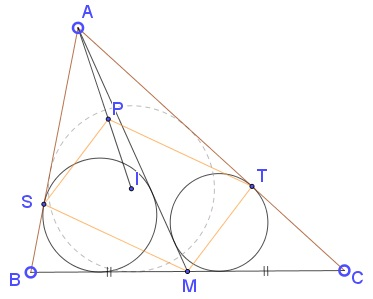# Thanos Kalogerakis' Collinearity in Triangle

### Source### Problem

$M$ is the midpoint of $BC$ in $\Delta ABC;$ $S$ is the intouch point on side $AB$ of $\Delta ABM;$ $T$ is the intouch point on side $AC$ of $\Delta ACM.$ Further, point $P$ inside $\Delta ABC$ satisfies $SP\parallel MT$ and $TP\parallel MS.$Prove that $A,P,I$ are collinear, where $I$ is the incenter of $\Delta ABC.$

### Solution

Draw two circle $\displaystyle w_1=C\left(M,\frac{a}{2}\right)$ and $\displaystyle w_2=C\left(A,AM-\frac{a}{2}\right).$ The two circles are tangent to each other at point $Z.$Let $D$ and $E$ be the point of intersection of $w_2$ with $AB$ and $AC,$ respectively. Then $S$ is the midpoint of $AD$ and $T$ is the midpoint of $BE.$ Indeed, in $\Delta ABM,$ $\displaystyle BS=\frac{1}{2}\left(\frac{a}{2}-AM+c\right)$ whereas $\displaystyle BD=c-\left(AM-\frac{a}{2}\right).$ Similarly, ET=CT.$By the converse of Varignon's theorem in quadrilateral$BDEC,P$coincides with the midpoint of$DE$and, since$\Delta ADE$is isosceles,$AP$also serves as the bisector of the angle at$A,\$ thus solving the problem.

### Acknowledgment

Thanos Kalogerakis has kindly communicated to me his problem along with a solution of his. He also pointed to a post by Reuben Dario at the Peru Geometrico facebook group where one can find additional solutions.